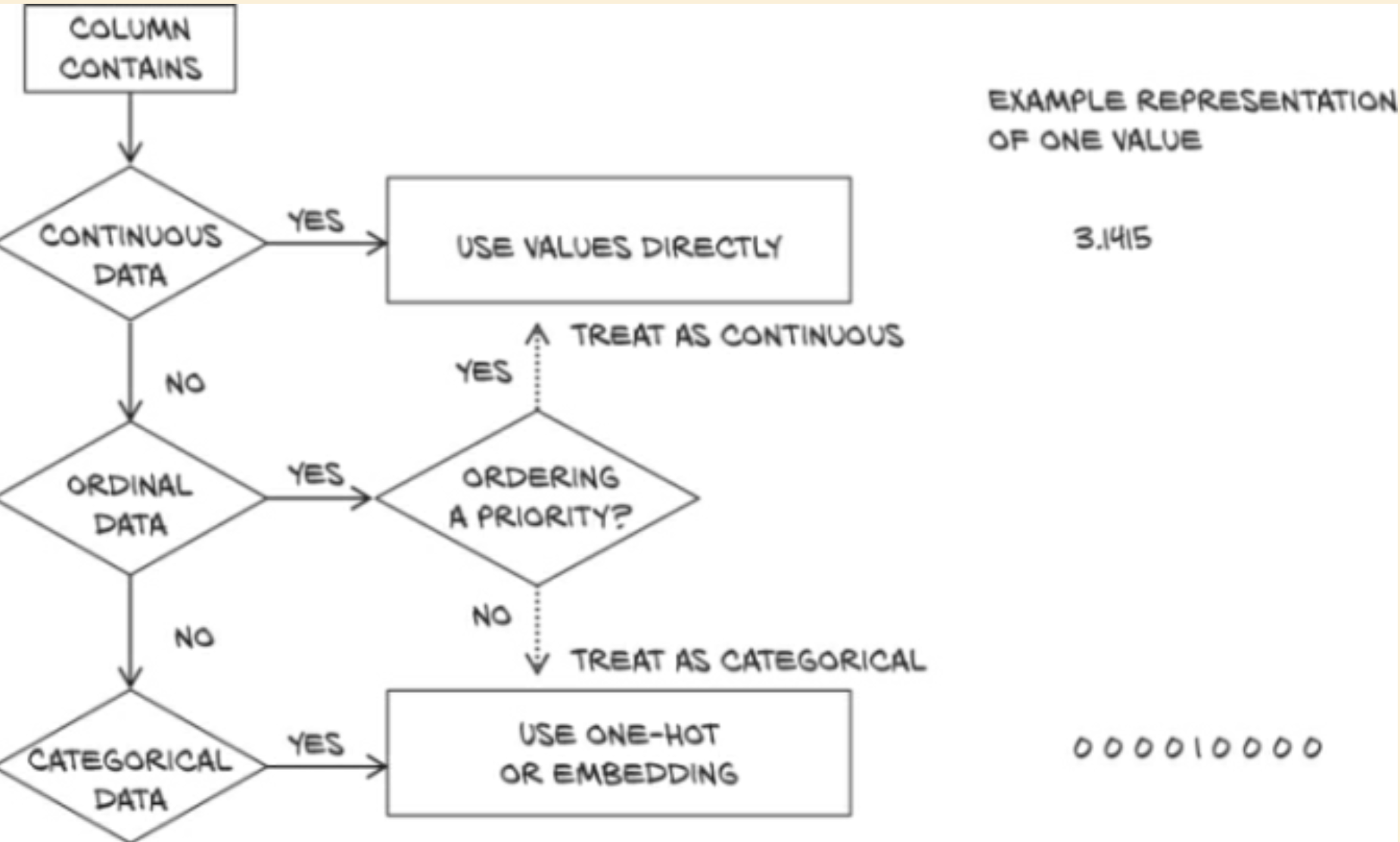# chapter 4 - Real-world Data Representation Using Tensors

Jan 01, 2023

## working with images

• accompanying ipython notebook
• imageio is a handy library for loading images into numpy arrays
• The book uses imageio v2 but they are preparing to release v3
• opening a single image is just as easy in v3
• I couldn't figure out how to open a whole directory of images in v3 the same way I could in v2 - see the notebook for details
• the book just chops alpha channels, which we've seen prior is a recurring problem for us
• Surely there's a better way to deal with this, like assume a white background and render to that?
• not gonna worry about it here though, they don't either
• torch assumes you have images arranged in (Channel, Height, Width) order while imageio returns (H, W, C)
• You can use permute to rotate the channels around cheaply
• preallocating and filling batches of images is less expensive than allocating on the fly
• Images are usually stored with uint8 R, G, B pixel values
• NNs work best with pixels in the range [0,1] or [-1,1], depending
• on what, they don't say
• normalization is tricky
• We can just divide by 255
• We also could compute the mean and standard deviation, and "scale it so that the output has zero mean and unit standard deviation across each channel"
• I can't say I really understand what this means
• In the book's example, we normalized a batch at a time
• In practice, it's a much better idea to normalize the entire dataset at once
• Volumetric data
• CT scan data is represented in grayscale, as a series of stacked 2d images
• raw data will often lack a channel dimension entirely, and be represented as (H, W, D)
• D == Depth
• We'll store it in batches as (N, C, D, H, W)
• Will tackle in much more detail in part 2

## tabular data

• notebook
• They say they prefer using pandas to load csv data - I'm glad they do it with numpy instead, to avoid introducing another library
• The data we have is a series of chemical measurements of wine, paired with a "quality" value as scored by wine tasters
• We could treat the quality as a continuous variable and regress on it, or treat it as a categorical value and try to guess it as a classification task
• either way, we want to pull it off of the rest of the input data
• One-hot encoding
• Instead of having the score we're trying to train towards be a number from 1 to 10, we can use one-hot encoding
• this means to have a 10-element array, with only one element being a 1, where each possible array maps to one possible label
• 1 maps to (1, 0, 0, 0, 0, 0, 0, 0, 0, 0)
• 2 maps to (0, 1, 0, 0, 0, 0, 0, 0, 0, 0)
• 3 maps to (0, 0, 1, 0, 0, 0, 0, 0, 0, 0)
• etc
• why?
• mapping quality scores to an integer induces an ordering on the scores
• appropriate in this case - but not always
• induces some sort of distance between scores - is 2 -> 4 really the same "disatnce" as 6 -> 8?
• If scores are purely discrete, one-hot encoding will be a better fit, as there's no implied distance
• one-hot encoding is also appropriate for quantitative scores when fractional values between integers scores make no sense for the application - when something is either this or that
• Can use the scatter_ method to generate a one-hot encoding
•• We can normalize our data by subtracting the mean and dividing by the standard deviation
• Why do we want to do this? Book says "it helps with the learning process" - why?
• see notebook for procedure
• T[B] will return only the indexes in T that are true in B